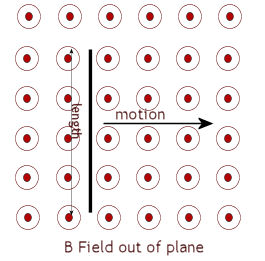### Magnetic Flux, Flux Density and Basic Electromechanical Energy Conversion

Magnetic flux, Φ is measured in Webers. Flux density, which is a measure of the amount of flux crossing a surface, is measured in Teslas. By definition 1 T = 1 Wb/m2.

We shall now examine two relationships between Mechanical energy and Electrical energy.

When a conductor of length, l, moves through a magnetic field of flux density, B, at rights angles to the field with velocity, v,  an emf , E,  is induced across the conductor. This emf is proportional to the flux density, conductor length and velocity of motion.

E = Blv

This basic principle allows the operation of electrical generators that convert Mechanical rotational energy to Electrical energy. We shall defer development of this concept until the lesson on generators and motors.Example: Calculate the emf induced across a conductor of length 2m when it moves with a constant speed of 0.5 m/s at right angles through a magnetic field of flux density 3 T.

Solution:

E = Blv = 3 × 2 × 0.5 = 3 V